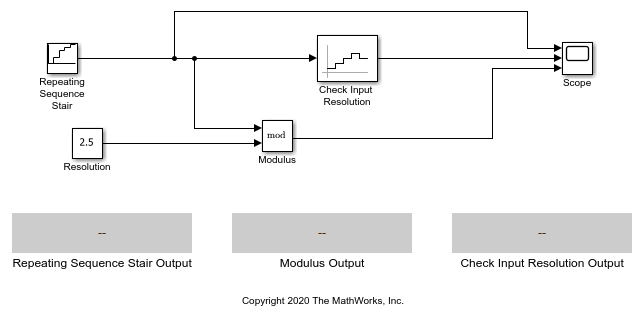# Check Signal Resolution with Check Input Resolution Block

You can use a Check Input Resolution block to check when a signal has a specified resolution. The block can help verify if a signal is quantized within specification, such as when checking the output of an analog-to-digital converter.In this example, a Repeating Sequence Stair block outputs the values [0 1 2 2.5 3] in sequence starting at `0`. During simulation, the block outputs each value in the vector for a time of 1.

First, the Check Input Resolution block calculates a modulus by calculating the remainder of the input value over the value of the Resolution parameter, which is set to `2.5`. To illustrate this calculation, this example also uses a Math Function block, labeled Modulus, with the Function parameter set to `mod`. The Modulus block takes the value of the Repeating Sequence Stair block over the value of a Constant block, labeled Resolution, which has the same value as the Resolution parameter.

Then the Check Input Resolution block checks if the modulus is smaller than a tolerance of `0.01`. If it is, the block asserts `true (1)`. Because the Output assertion signal parameter of the Check Input Resolution Block is selected, the block outputs the assertion value. Run the simulation to observe the model output, or use the Step Forward button to step through each step.When you run the model, the Scope block plots the Repeating Sequence Stair block output, the Modulus block output, and the Check Input Resolution block output. If you use the Step Forward button, the data populates the three Display blocks at each time step.

1. Initially, the modulus of the Repeating Sequence Stair block over the resolution is `0`, so the Check Input Resolution block outputs `1`.

2. When the simulation time reaches `1`, the Repeating Sequence Stair block outputs `1`. The modulus rises to `1`, which is larger than the tolerance, causing the Check Input Resolution block to output `0`.

3. The assertion stays at `0` until the time is `3`, when the Repeating Sequence Stair block outputs `2.5`. The modulus drops to `0`, and the assertion returns to `1`.

4. Finally, the Repeating Sequence Stair block outputs `3` when the time is `4`, which causes the assertion to be `1`.

The model repeats this pattern until the simulation end time.